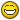# Table for counting Plines length in layers

## Recommended PostsAhoy mates!

I need help. i found this lisp online and im still too noob to read and understand how it goes properly. also i want to make a change in it but im not sure how..

Lisp:

```(defun C:LAYLENGTH ( / *error* acdoc ss p i e a d l) (vl-load-com)
(vla-startundomark acdoc)

(defun *error* (msg)
(and
msg
(not (wcmatch (strcase msg) "*CANCEL*,*QUIT*,*BREAK*,*EXIT*"))
(princ (strcat "\nError: " msg))
)
(if
(= 8 (logand (getvar 'undoctl) 8))
(vla-endundomark acdoc)
)
(princ)
)

(if
(and
(setq ss (ssget ":L" '((0 . "LINE,POLYLINE,LWPOLYLINE,ARC,CIRCLE,ELLIPSE,SPLINE"))))
(setq p (getpoint "\nTable scale depend on annotation scale.\nSpecify table insert point: "))
)
(progn
(repeat
(setq i (sslength ss))
(setq e (ssname ss (setq i (1- i)))
a (cdr (assoc 8 (entget e)))
d (vlax-curve-getdistatparam e (vlax-curve-getendparam e))
)
(if
(setq o (assoc a l))
(setq l (subst (list a (+ (cadr o) d)) o l))
(setq l (cons (list a d) l))
)
)
(setq l (vl-sort l '(lambda (a b) (< (car a) (car b)))))
(insert_table l p)
)
)
(*error* nil)
(princ)
)

(defun insert_table (lst pct / tab row col ht i n space)
(setq space (vlax-get acDoc (if (= 1 (getvar 'cvport)) 'PaperSpace 'ModelSpace))
ht  (/ 2.5 (cond ((getvar 'cannoscalevalue)) (1.0)))
pct (trans pct 1 0)
n   (trans '(1 0 0) 1 0 T)
tab (setq tab (vla-addtable space (vlax-3d-point pct) (+ 2 (length lst)) (length (car lst)) (* 2.5 ht) ht))
)
(vlax-put tab 'direction n)

(mapcar
(function
(lambda (rowType)
(vla-SetTextStyle  tab rowType (getvar 'textstyle))
(vla-SetTextHeight tab rowType ht)
)
)
'(2 4 1)
)

(vla-put-HorzCellMargin tab (* 0.14 ht))
(vla-put-VertCellMargin tab (* 0.14 ht))

(setq lst (cons (mapcar '(lambda (a) (strcat "Col" (itoa (1+ (vl-position a (car lst)))))) (car lst)) lst))

(setq i 0)
(foreach col (apply 'mapcar (cons 'list lst))
(vla-SetColumnWidth tab i
(apply
'max
(mapcar
'(lambda (x)
((lambda (txb) (+ (abs (- (caadr txb) (caar txb))) (* 2.0 ht)))
(textbox (list (cons 1 (vl-princ-to-string x)) (cons 7 (getvar 'textstyle)) (cons 40 ht)))
)
)
col
)
)
)
(setq i (1+ i))
)

(setq lst (cons '("TITLE") lst))

(setq row 0)
(foreach r lst
(setq col 0)
(vla-SetRowHeight tab row (* 1.5 ht))
(foreach c r
(vla-SetText tab row col (if (numberp c) (rtos c) (vl-princ-to-string c)))
(setq col (1+ col))
)
(setq row (1+ row))
)
)```

This lisp wants me to select what i want it to count. which is nice and all and workable.. and then places a table where i choose in which it shows me the combined length of what i picked by layers

but Id rather have it automatically call out on specific list of layers in which to count ONLY its Lines, Plines, 2D Plines and 3D Plines  LENGTH combined seperated by layers.

is it possible?

example name of a layer is MUG_6602

i have atleast 8 layers which isnt always active but always appear in the dwg and never frozen or hidden.

I'd also rather it to show that if there are no Plines so it would return a 0 value in the table.

and also if i can add another line in this post and push it to the edge, id like the table to update automatically like when using the UPDATE command on fields

Im asking too much.. i know it.. but it could really make me look good in my companyThnx in advance to u all

Edited by Mugna101
Specified my needs more,,

##### Share on other sitesTry this

`(setq ss (ssget '((0 . "LINE,POLYLINE,LWPOLYLINE,ARC,CIRCLE,ELLIPSE,SPLINE")(8 . "0,red")))) ; add your layer names`

•1

##### Share on other sites15 hours ago, BIGAL said:

Try this

```
(setq ss (ssget '((0 . "LINE,POLYLINE,LWPOLYLINE,ARC,CIRCLE,ELLIPSE,SPLINE")(8 . "0,red")))) ; add your layer names```

SUPERB! tested it quickly and it worked like a charm!

Edited by Mugna101
My reply was in the quote.. got it out

## Join the conversation

You can post now and register later. If you have an account, sign in now to post with your account.
Note: Your post will require moderator approval before it will be visible.×   Pasted as rich text.   Paste as plain text instead

Only 75 emoji are allowed.